瓜子大数据架构首曝光：掩藏在“二手车”背后的技术思考

6 月 9 日 AI前线AI 前线导读：“瓜子二手车直卖网，没有中间商赚差价”，这句深入人心的广告语掩盖了这家公司的技术实力和探索。据悉，这是车好多大数据技术团队第一次对外技术采访，浅谈大数据架构及对云平台大数据服务选型的思考，有共性也有差异，个中原因值得探讨。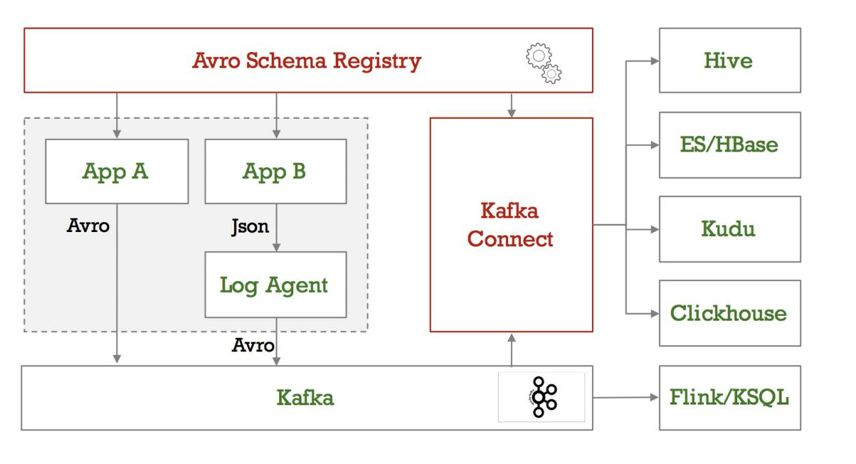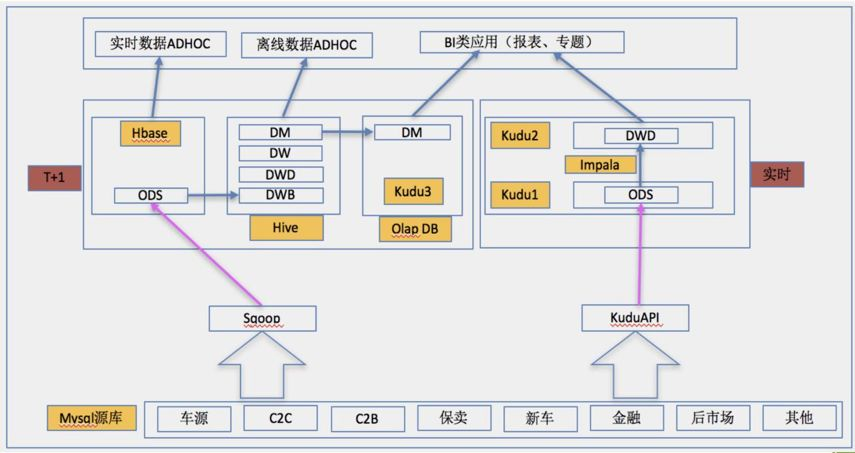今日荐文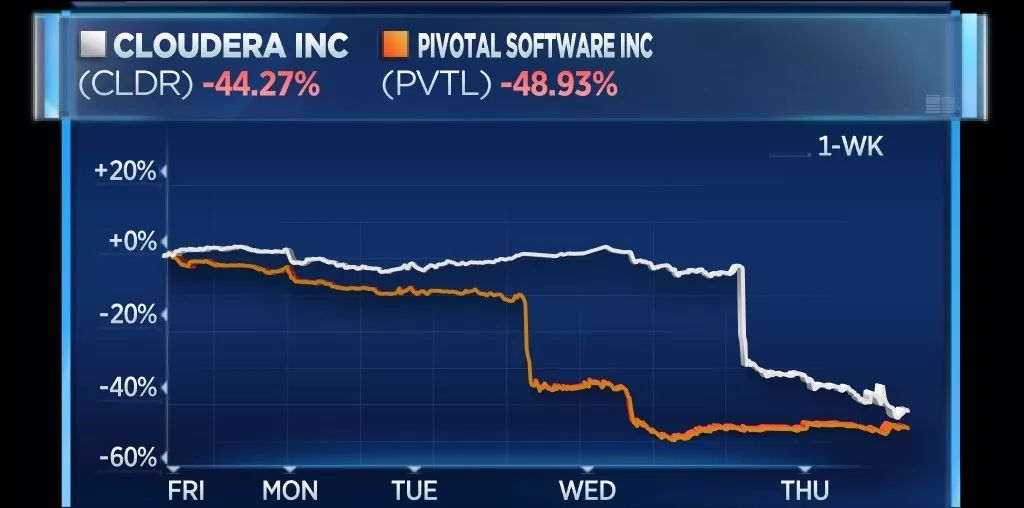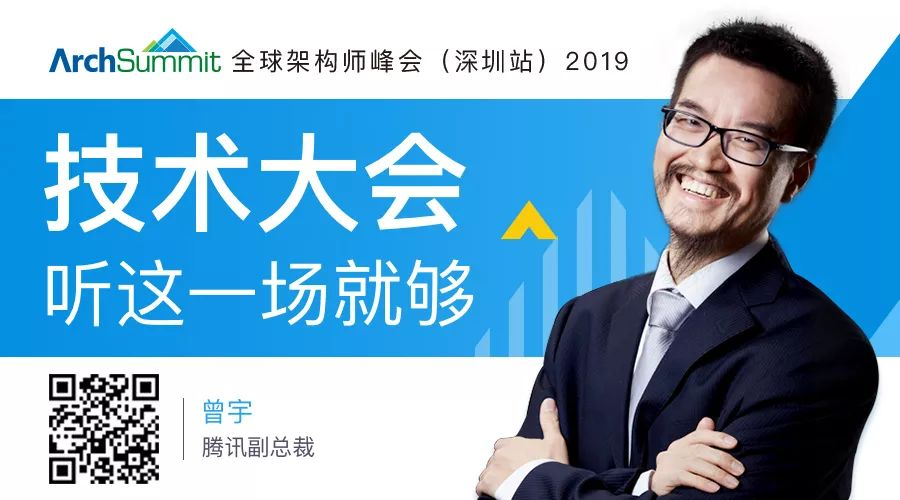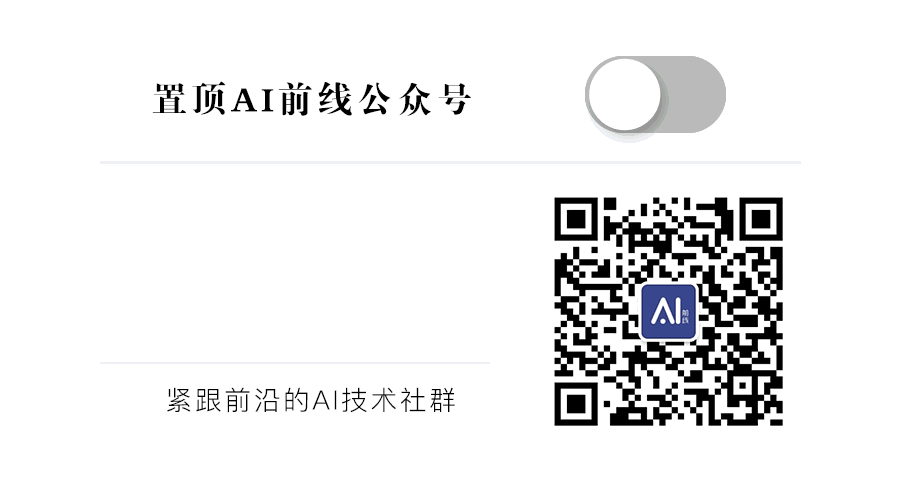0+1+

相关内容0+0+0+0+0+0+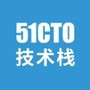0+0+0+0+0+0+0+0+0+0+0+0+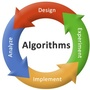0+0+0+0+0+0+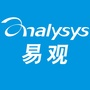0+1+0+0+0+0+
Top